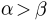# Writing mathematical expressions¶

An introduction to writing mathematical expressions in Matplotlib.

You can use a subset TeX markup in any matplotlib text string by placing it inside a pair of dollar signs ($). Note that you do not need to have TeX installed, since Matplotlib ships its own TeX expression parser, layout engine, and fonts. The layout engine is a fairly direct adaptation of the layout algorithms in Donald Knuth's TeX, so the quality is quite good (matplotlib also provides a usetex option for those who do want to call out to TeX to generate their text (see Text rendering With LaTeX). Any text element can use math text. You should use raw strings (precede the quotes with an 'r'), and surround the math text with dollar signs ($), as in TeX. Regular text and mathtext can be interleaved within the same string. Mathtext can use DejaVu Sans (default), DejaVu Serif, the Computer Modern fonts (from (La)TeX), STIX fonts (with are designed to blend well with Times), or a Unicode font that you provide. The mathtext font can be selected with the customization variable mathtext.fontset (see Customizing Matplotlib with style sheets and rcParams)

Here is a simple example:

# plain text
plt.title('alpha > beta')


produces "alpha > beta".

Whereas this:

# math text
plt.title(r'$\alpha > \beta$')


produces "".

Note

Mathtext should be placed between a pair of dollar signs ($). To make it easy to display monetary values, e.g., "$100.00", if a single dollar sign is present in the entire string, it will be displayed verbatim as a dollar sign. This is a small change from regular TeX, where the dollar sign in non-math text would have to be escaped ('\$'). Note While the syntax inside the pair of dollar signs ($) aims to be TeX-like, the text outside does not. In particular, characters such as:

`

## Example¶

Here is an example illustrating many of these features in context.

Keywords: matplotlib code example, codex, python plot, pyplot Gallery generated by Sphinx-Gallery